A Flash Developer Resource Site

# Thread: how to draw rounded Rectangle

1. ## how to draw rounded Rectangle

hello all

how to draw rounded Rectangle like this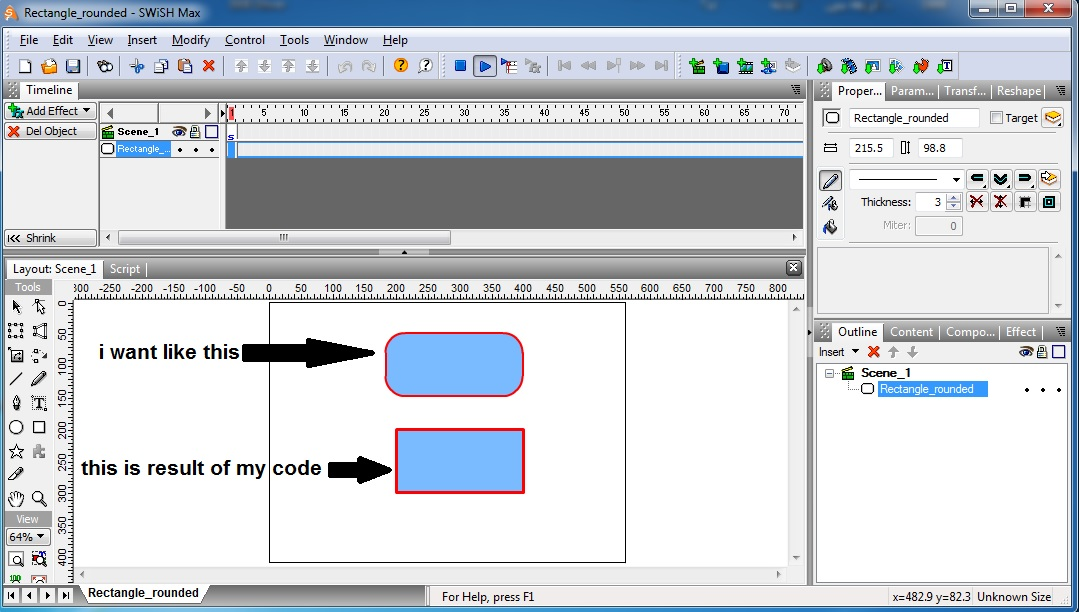my code is :

PHP Code:
``` onFrame (1) {    function createRectangle(w:Number, h:Number, bgColor:Number,stroke:Number,x:Number, y:Number,):MovieClip { this.createEmptyMovieClip("mc",this.getNextHighestDepth()); mc.lineStyle(5, stroke, 100, true, "round", "round", "round", 8); mc.beginFill(bgColor); mc.lineTo(w, 0); mc.lineTo(w, h); mc.lineTo(0, h); mc.lineTo(0, 0); mc._x = x; mc._y = y; return mc; }  createRectangle(200, 100, 0x7ABAFF,0xFF0000,200,200); }  ```

swishmax file : https://app.box.com/s/2tang7dsl13hid9emosffig7hfub46lg

thanks for helpReply With Quote

2. Hello,

At the onFrame(2) scripting section you can set x, y, width, height, color, and set the rectangle options percent to be 0-100 as well.
PHP Code:
``` createRectangle(0,0,500,100,0x370640,"100%");//x,y,width,height,color,rectangle options. createRectangle(150,150,100,100,0x370640,"50%");//x,y,width,height,color,rectangle options.  ```
PHP Code:
``` onFrame(2){ stop(); var size=0//Temporary data for rectangle options. var offset_x=0 var offset_y=square._height var curve_size=100 var size2=0//Temporary data for rectangle options. var offset_x2=0 var offset_y2=0 var curve_size2=0//60-100 var size3=0//Temporary data for rectangle options. var offset_x3=square._width var offset_y3=square._height var curve_size3=0//60-100 var size4=0//Temporary data for rectangle options. var offset_x4=square._width var offset_y4=0 var curve_size4=0 var rect=0//Rect increases after each createRectangle() function call to create mc0, mc1, mc2 etc... function createRectangle(x,y,w,h,color,rectangle_options) {//Create a rectangle. this.createEmptyMovieClip("mc"+rect,this.getNextHighestDepth());//Create empty movieclip with name "mc"+rect. rectangle_options=int(rectangle_options.split("%"))//Remove the percent string from the number & convert from string to int. if(rectangle_options>100){//Condition for preventing the variable from exceeding 100. rectangle_options=100//Set to 100% if rectangle_options is greater than 100. } var percent=rectangle_options//Create a variable called percent to equal rectangle_options. if(percent<1){//If percent is less than 1 make sure to use 0.1 so the beginFill() can occur. percent=0.1 } if(w>h){//If width is greater than height, use the smallest number to create the curve. rectangle_options=(((h/100)*percent)/2) }else{ rectangle_options=(((w/100)*percent)/2)//Use the smallest between width & height when setting a rectangle option. } curve_size=rectangle_options//Set the curve size for each face. curve_size2=rectangle_options curve_size3=rectangle_options curve_size4=rectangle_options offset_x=x offset_y=y+h offset_x2=x offset_y2=y offset_x3=x+w offset_y3=y+h offset_x4=x+w offset_y4=y _root["mc"+rect].clear()//Clear any previous linestyle graphics. _root["mc"+rect].lineStyle(2, color, 100, true, "round", "round", "round", 8);//Set the line style thickness. _root["mc"+rect].beginFill(color)//Set the color fill. _root["mc"+rect].moveTo(  -(0+(-offset_x)+(-curve_size))    ,     - (0-offset_y)    );//Draw bottom left curve. _root["mc"+rect].curveTo(   (size+offset_x)    , ((size/(-curve_size))+offset_y)      ,      (size+offset_x) ,   (-size+offset_y+(-curve_size))) _root["mc"+rect].moveTo(0+offset_x2+curve_size2,0+offset_y2);//Draw top left curve. _root["mc"+rect].curveTo(size2+offset_x2,(size2/curve_size2)+offset_y2,size2+offset_x2,-size2+offset_y2+curve_size2) _root["mc"+rect].moveTo(0+offset_x3-curve_size3,0+offset_y3);//Draw bottom right curve. _root["mc"+rect].curveTo(size3+offset_x3,(size3/-curve_size3)+offset_y3,size3+offset_x3,-size3+offset_y3-curve_size3) _root["mc"+rect].moveTo(  -(0+(-offset_x4)+curve_size4)    ,     - (0-offset_y4)    );//Draw top right curve. _root["mc"+rect].curveTo(   (size4+offset_x4)    , ((size4/curve_size4)+offset_y4)      ,      (size4+offset_x4) ,   (-size4+offset_y4+curve_size4)) _root["mc"+rect].moveTo(-(0+(-offset_x)+(-curve_size))  ,y);//TOP LINE between the curves. _root["mc"+rect].lineTo( -( (-(0+(-offset_x)+(-curve_size)) ) - -(0+(-offset_x4)+curve_size4))  +-(0+(-offset_x)+(-curve_size)) ,y) _root["mc"+rect].moveTo(-(0+(-offset_x)+(-curve_size))  , (size/(-curve_size))+offset_y);//BOTTOM LINE _root["mc"+rect].lineTo( -( (-(0+(-offset_x)+(-curve_size)) ) - -(0+(-offset_x4)+curve_size4))  +-(0+(-offset_x)+(-curve_size)) ,((size/(-curve_size))+offset_y)) _root["mc"+rect].moveTo(x,  (-size4+offset_y4+curve_size4))//LEFT LINE between the curves. _root["mc"+rect].lineTo(x,  (-size+offset_y+(-curve_size))-(-size4+offset_y4+curve_size4)+(-size4+offset_y4+curve_size4)) _root["mc"+rect].moveTo(x+w,  (-size4+offset_y4+curve_size4))//RIGHT LINE between the curves. _root["mc"+rect].lineTo(x+w,  (-size+offset_y+(-curve_size))-(-size4+offset_y4+curve_size4)+(-size4+offset_y4+curve_size4)) _root["mc"+rect].beginFill(color) _root["mc"+rect].moveTo(-(0+(-offset_x)+(-curve_size))  ,y);//Octogon graphic to fill the other section. _root["mc"+rect].lineTo( -( (-(0+(-offset_x)+(-curve_size)) ) - -(0+(-offset_x4)+curve_size4))  +-(0+(-offset_x)+(-curve_size)) ,y) _root["mc"+rect].lineTo(x+w,  (-size4+offset_y4+curve_size4)) _root["mc"+rect].lineTo(x+w,  (-size+offset_y+(-curve_size))-(-size4+offset_y4+curve_size4)+(-size4+offset_y4+curve_size4)) _root["mc"+rect].lineTo( -( (-(0+(-offset_x)+(-curve_size)) ) - -(0+(-offset_x4)+curve_size4))  +-(0+(-offset_x)+(-curve_size)) ,((size/(-curve_size))+offset_y)) _root["mc"+rect].lineTo(-(0+(-offset_x)+(-curve_size))  , (size/(-curve_size))+offset_y); _root["mc"+rect].lineTo(x,  (-size+offset_y+(-curve_size))-(-size4+offset_y4+curve_size4)+(-size4+offset_y4+curve_size4)) _root["mc"+rect].lineTo(x,  (-size4+offset_y4+curve_size4)) rect++ return mc } createRectangle(0,0,500,100,0x370640,"100%");//x,y,width,height,color,rectangle options. createRectangle(150,150,100,100,0x370640,"50%");//x,y,width,height,color,rectangle options. }  ```Reply With Quote

3. thanks my friend.

Actually I didn't understand this code because it's complicated

Can you make a loop to create 3 squares like in this pictureI want :

1- Randomize the colors ( red . blue . green ) of these squares each time

2- Add text in each square and this text loads variables from external txt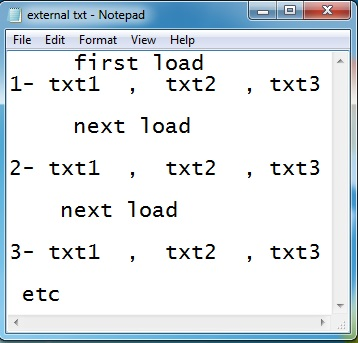3- when i press the ( right value = txt3 ) ===> go to next loadReply With Quote

4. Hi, this answer is for the loop & line style.

At the onFrame(2) scripting section, you can call this at the bottom:
PHP Code:
``` var colors:Array=[0xFF0000,0x00FF00,0x0000FF]//This list stores the 3 colors for the for loop. for(var i=0;i<3;i++){//This is the for loop that will go up to 3 for the colors array above. var mc = createRectangle(0,0,400,100,colors[i],0xFFFF33,"50%",3);//x,y,width,height,color,line color,rectangle options, stroke size.//var mc gets returned from createRectangle to store the movieclip you just created. mc._y=mc._height*i+(5*i)//Use mc._x & mc._y to reposition the movieclip you just created with createRectangle. }  ```
Here is another example:
PHP Code:
``` createRectangle(0,0,400,100,0x000000,0xFFFF33,"50%",3); //For the first 2 parameters, x & y will be at 0 in the container, It is better to use 0,0 for x & y since you want to access it as a movieclip. var mc = createRectangle(0,0,400,100,0x000000,0xFFFF33,"50%",3);//Using var mc = createRectangle you can move the rectangle around as mc._x=50 or mc._y=50 //The correct way to access mc's true name is using: _root["mc0"]._x=50 for the first square, _root["mc1"]._x=100 for the second square & so on. //You can adjust the true width and height of the squares by modifying 400 for the width & modifying 100 for the height. //0x000000 is used as the fill color. //0xFFFF33 is used as the line color/stroke color. //You can adjust the curve of the square by modifying 50% to 100% for max curve or 0% for no curve. //You can modify the line size/stroke size by adjusting the last value which is 3.  ```Reply With Quote

5. that is great

can you add rondom _y positionReply With Quote

6. Hello,

At the onFrame(2) scripting section for the function called createRectangle() I added these lines:
PHP Code:
``` _root["mc"+rect].createTextField("num"+0,mc.getNextHighestDepth(),0,0,_root["mc"+rect]._width,_root["mc"+rect]._height/1.5);//Create textfield inside of current round square. _root["mc"+rect]["num"+0]._x=0//Set the x coordinate to be 0 since the size of the textfield will be the size of the round squares width. _root["mc"+rect]["num"+0]._y=int(_root["mc"+rect]._height/2-(_root["mc"+rect]["num"+0]._height/2))//Initial y coordinate _root["mc"+rect]["num"+0].border = false;//Hide border style. _root["mc"+rect]["num"+0].text = "Hello "+rect;//Initial text data. _root["mc"+rect]["num"+0].selectable=false//Make the textfield non-selectable to avoid interfering with clicking the square as a button. myformat = new TextFormat();//Create  a new global variable for myformat. myformat.size=_root["mc"+rect]._height/2 ;//Set the size of the text to be half the height of the round square. myformat.color = 0xFFFFFF//Set the color of the text to be white. myformat.bold=true;//Set the textfields style to bold. myformat.align="center";//Center the text field. _root["mc"+rect]["num"+0].setTextFormat(myformat);//Apply the textformat style to the current textfield. _root["mc"+rect]["num"+0]._height=_root["mc"+rect]["num"+0].textHeight+_root["mc"+rect]["num"+0].textHeight/10 //Create a suitable height for the textfield which is textHeight + textHeight/10 for the small spacing over the text that will cut off the bottom. _root["mc"+rect]["num"+0]._y=((_root["mc"+rect]._height/2-_root["mc"+rect]["num"+0].textHeight/2)-_root["mc"+rect]["num"+0].textHeight/30)-((_root["mc"+rect]["num"+0].textHeight/10)/2)//Set a _y centering based on the math above. _root["mc"+rect].blendMode="layer"//Set the blend mode of the square to layer so that adjusting the alpha of the round square will look proper. _root["mc"+rect].position=0//Save the initial position of the textfield to be 0 for the current round square. _root["mc"+rect].onRelease=function(){//Convert the current round square to a button. this.position++//Increase the position variable of the current square. this["num"+0].text=_root.text_array_1[this._name.split("c")].split(",")[this.position+1]//Modify the text of the current square when it is pressed. this["num"+0].setTextFormat(myformat);//Update the textformat settings for the current round square. }  ```

At the onFrame(2) scripting section near the end, the program will load "settings.txt":
PHP Code:
``` var load_vars_1 = new LoadVars();//Create a new load vars.                 var text_array_1 = new Array();//Create an array to store the loaded data. load_vars_1.load("settings.txt");//Load the settings.txt load_vars_1.onData = function(e) {//Accessor for settings.txt data is:    e.split("\n")[ 0 ].split("\r")  ,   e.split("\n")[ 1 ].split("\r")   etc... for(var i=0;i<e.split("\n").length;i++){//Check length of new line character loaded from settings.txt text_array_1.push(e.split("\n")[i].split("\r"))//Push each line of data that was loaded from the settings.text file to the variable called text_array_1.    _root["mc"+i]["num"+0].text=text_array_1[i].split(",")[_root["mc"+0].position+1]//Set the text field for each round square to say the proper data. _root["mc"+i]["num"+0].setTextFormat(myformat);//Set text format when updating text fields. } }       randomizeArray()//Randomize the color array at the top of the program. for(var i=0;i<3;i++){ var mc = createRectangle(0,0,500,100,colors[i],0xFFFF33,"50%",2);//x,y,width,height,color,line color,rectangle options. mc._y=mc._height*i+(5*i)//Set the y coordinate for each round square created. }  ```Reply With Quote

7.Reply With Quote

8. 1- can you load data arrangement as ( txt1,txt2,txt3 )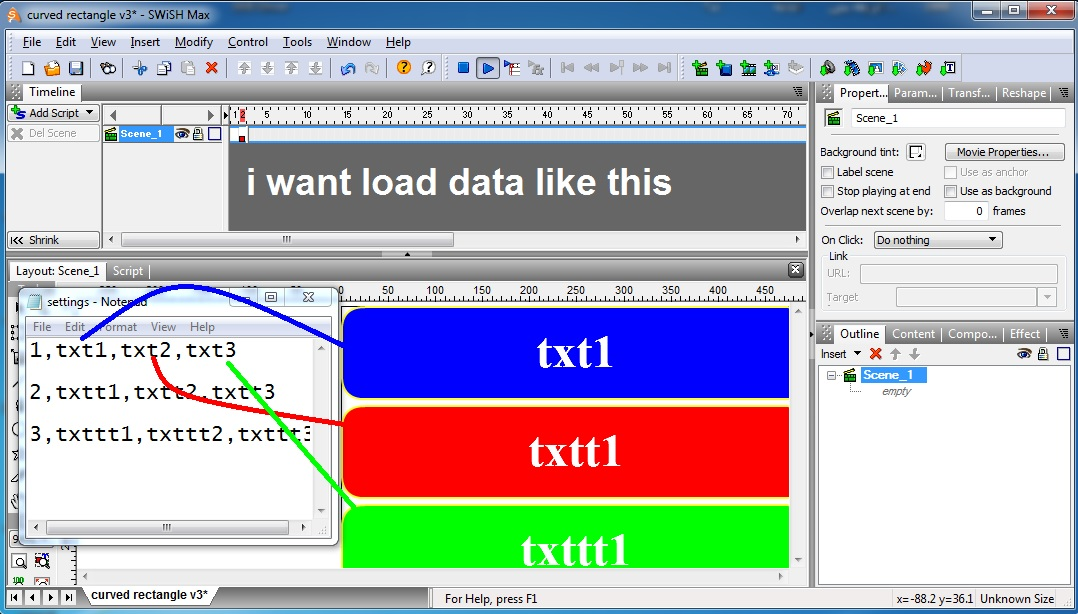2- the defult true value=the third value (txt3) . it means when i press it==> score text +=1

in this case the green button is the true value

can you make load rondom ( txt1,txt2,txt3 )

3- can you add score text and if i press (txt3 ) ==>score text +=1

but if i press ( txt1) or (txt2) ==> go to next load

thanks for helpReply With Quote

9. Hello,

The score text is stored in this.position for each button:
PHP Code:
``` var load_vars_1 = new LoadVars();                         var text_array_1 = new Array(); load_vars_1.load("settings.txt");   load_vars_1.onData = function(e) { for(var i=0;i<e.split("\n").length;i++){ text_array_1.push(e.split("\n")[i].split("\r"))       _root["mc"+i]["num"+0].text=text_array_1.split(",")[i+1] + " score text=" + _root["mc"+i].position //The start up load is at array position 0: text_array_1[  0  ].split(",")[i+1] passed the first comma. _root["mc"+i]["num"+0].setTextFormat(myformat); } }  ```
At the bottom of the function called createRectangle() in the onFrame(2) scripting section:
PHP Code:
``` _root["mc"+rect].onRelease=function(){//On release function for the round square. if(this.position<_root.text_array_1.length-1){//If this buttons position is smaller than the text_array that stores the settings.txt data. this.position++//We can still increase the number for the current button. this["num"+0].text=_root.text_array_1[this.position].split(",")[int(this._name.split("c"))+1] + " score text=" + this.position //Set the textfield for the current round square to be:   _root.text_array_1[   this.position   ].split(",")[int(this._name.split("c"))+1] //this.position to go to the second array spot & split mc2 to be +1 for the current array. this["num"+0].setTextFormat(myformat);//Update the textformat style. } }  ```Reply With Quote

10. Excuse me, I am unable to explain exactly what is required because my bad English

this pic explains what is required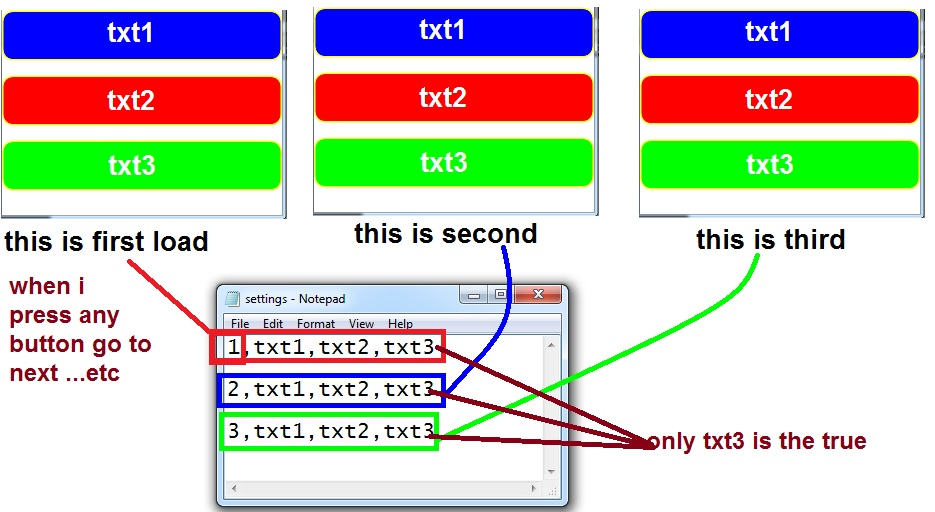Reply With Quote

11. Hi, for v5 it will do for all buttons.Reply With Quote

12. yes thisReply With Quote

13. Hello, the colors load up randomly as well as the order of the selections.

On line 234 of the onFrame(2) scripting section:
PHP Code:
``` randomizeArray(colors)//Randomizes the array called colors.  ```
On lines 216 of the onFrame(2) scripting section inside of the load complete event of the settings.txt file the data is loaded in the first loop and in the second loop each array gets transferred into the temp_array to be randomized and pushed back into the text_array_1:
PHP Code:
``` var load_vars_1 = new LoadVars();                         var text_array_1 = new Array(); load_vars_1.load("settings.txt");   load_vars_1.onData = function(e) { for(var i=0;i<e.split("\n").length;i++){ text_array_1.push(e.split("\n")[i].split("\r"))//Push each line from the loaded text file into an array position. _root["mc"+i]["num"+0].text=text_array_1.split(",")[i+1]  _root["mc"+i]["num"+0].setTextFormat(myformat); } for(var i=0;i<text_array_1.length;i++){//Loop through each line of data in the array that was loaded from the textfile. var temp_array=[]//Create a temporary array to randomize. for(var j=1;j<text_array_1[i].split(",").length;j++){//Skip the  1-, 2- etc... by starting the array at 1 and looping through the amount of comma's per line. temp_array.push(text_array_1[i].split(",")[j])//Push each comma split array element into the temporary array to randomize in the next line. } text_array_1[i]=text_array_1[i].split(",")+","+randomizeArray(temp_array)+","+String(text_array_1[i].split(","))//The current line of data that was from the text file will now start with 1-, 2- etc... + randomize the temp array + include the true answer at the end apart from the version that was randomized. } for(var i=0;i<text_array_1.length;i++){//Show a random order for the initial startup. _root["mc"+i]["num"+0].text=text_array_1[_root["mc"+i].position].split(",")[i+1]  _root["mc"+i]["num"+0].setTextFormat(myformat); } }  ```Reply With Quote

14. thank you very muchReply With Quote

15.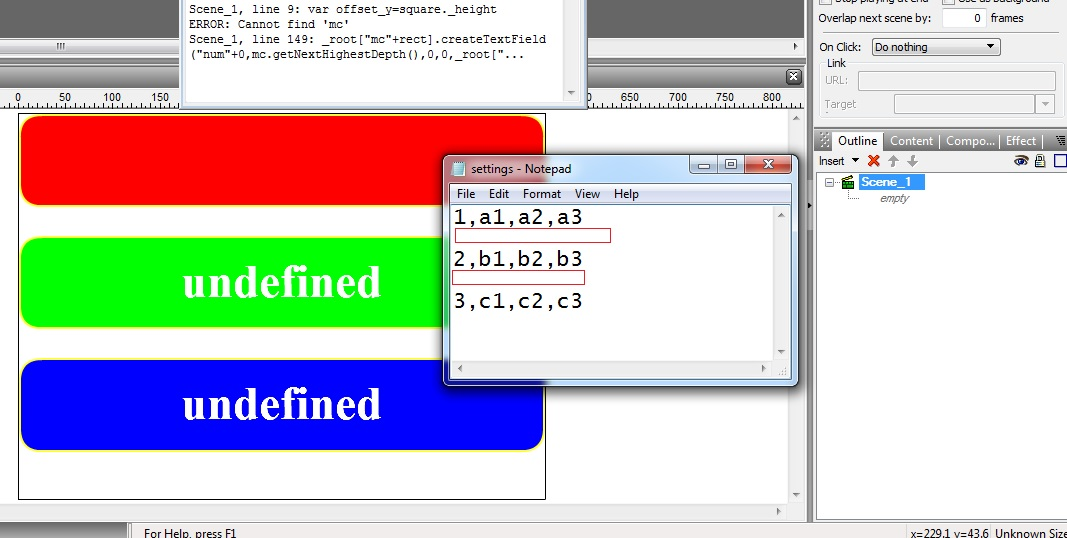when i add space between 1 and 2 and 3 in my text ====> undefined value

can you solve this error

can you load rondom from my text
this means : if this is first load may be 1 or 2 or 3 etcReply With Quote

16. Hi,

At the onFrame(2) scripting section for line 237 you can just call randomArray() on text_array_1
PHP Code:
``` randomizeArray(text_array_1)//The array called text_array_1 stores each line in an array spot as a string. //1,txt1,txt2,txt3    <-------- text_array //2,txtt1,txtt2,txtt3 <-------- text_array //3,txttt1,txttt2,txttt3 <-------- text_array //And now randomized with randomizeArray(text_array_1)  ```
At the onFrame(2) scripting section you can use the function called trimText() to fix any extra spacing for the loading "settings.txt".
PHP Code:
``` function trimText(e){ var tempstr="" _root.data_field.text="hi" for(var i=0;i<e.split("\n").length;i++){ if(e.split("\n")[i]!="\r"){ tempstr+=(e.split("\n")[i])+"\n" } } tempstr=tempstr.substr(0,tempstr.length-1) return tempstr }  ```
You can see at the onData function for the loaded text file that any errors in the spacing can be fixed with this line:
PHP Code:
``` e=_root.trimText(e)//Fix spacing issues.  ```Reply With Quote

17. Hello, I put randomize colors for each button press.

On lines 168:
PHP Code:
``` randomizeArray(colors)//Randomize the order of the colors. for(var i=0;i<text_array_1.length;i++){ updateRectangle(0,0,Stage.width,100,colors[i],0xFFFF33,"50%",3,_root["mc"+i]);//Update the rectangle colors with the new order. }  ```Reply With Quote

18. Better randomizing:Reply With Quote

19. professionalReply With Quote

####Posting Permissions

• You may not post new threads
• You may not post replies
• You may not post attachments
• You may not edit your posts
•

 » Home » Movies » Tutorials » Submissions » Board » Links » Reviews » Feedback » Gallery » Fonts » The Lounge » Sound Loops » Sound FX » About FK » Sitemap